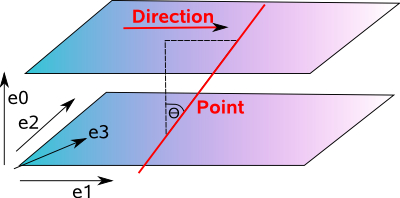# Maths - Projective Space using Geometric Algebra

We can represent rotations by using a combination of a 3 dimensional bivector and the real axis, this is equivalent to quaternions. Imagine that the four dimensions are all drawn perpendicular to each other, this is a bit difficult to draw in 2 dimensions, so we will crunch the 3 bivector dimensions together for ease of drawing:On this diagram a straight line through the origin contains all the 4D points representing the same rotation. The red line below might represent, for example, 90° about the y axis.

As we move along the line we get different scaling factors.

If m = w + x e23 + y e31 + z e12

where:

• m = a 3D multivector
• w,x,y,z = normalised coordinates of the quaternion

then α q = α w + α x e23 + α y e31 + α z e12

where:

• α = a scalar scaling factor, the actual scaling factor is α²

This scaling factor does not effect the rotation, only the magnitude of the output.

If α = 1, that is no scaling just pure rotation, that will be represented by the red circle on the diagram above.

We can see from the diagram that there are two places, representing two multivectors, that the red line and the red circle intersect. So there are two multivectors that represent every rotation, we know that from quaternion pages.

### Isometrys

So far we can only so rotation and scaling, what we want to do is rotation and translation.

We haven't yet used the vector (e1,e2 and e3) dimensions, it would be really nice if we could use it to represent translation, unfortunately we would have to combine translations using the addition operator but we combine rotations using the rotation operator which makes it difficult to combine translation and rotation. We can't combine translations by multiplications because vector times vector is a bivector not a vector?

We will therefore try to make translation more like rotation as follows: add a fourth dimension (e0) which is mutually perpendicular to the other dimensions.This representation also has the advantage of distinguishing between the representation of a point and a vector.

• A point is represented by a line through the origin
• A vector (direction and magnitude) is represented by a line in the e0=1 plane.

Where I can, I have put links to Amazon for books that are relevant to the subject, click on the appropriate country flag to get more details of the book or to buy it from them.Geometric Algebra for Computer Science: An Object-oriented Approach to Geometry. This book stresses the Geometry in Geometric Algebra, although it is still very mathematically orientated. Programmers using this book will need to have a lot of mathematical knowledge. Its good to have a Geometric Algebra book aimed at computer scientists rather than physicists. There is more information about this book here.